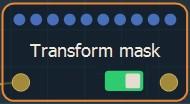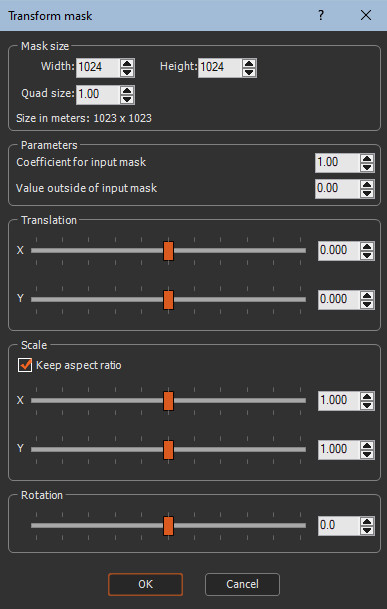This node modifies the first mask before it is inserted into a second mask.Double click on the node to open its parameters:### Editing a Transform mask node

Edit the Transform mask node in the parameters dialog:

• Coefficient for the input mask: Sets the coefficient by which the height of the input mask is multiplied.
• Height outside of the input: Sets the height of the output mask vertices around the input mask If the output mask is larger than the input mask.
• Translation X and Y: Translates the input mask along the X and Y axis. The translation values are relative to the output mask size:
• 0 sets the center of the input mask to the center of the output mask.
• -1 sets the center of the input mask to the left border of the output mask.
• 1 sets the center of the input mask to the right border of the output mask.
• Scale X and Y: Scales the input mask along the X and Y axis.
•  Check Keep aspect ratio to scale along the X or Y scale preserve the relative horizontal and vertical sizes.Note that when the scale manipulator is displayed, it is no longer possible to select a single axis; both axes are selected at the same time.
• Rotation: Rotates the input mask around its center from 0 to 180 degrees.

Parameter Use